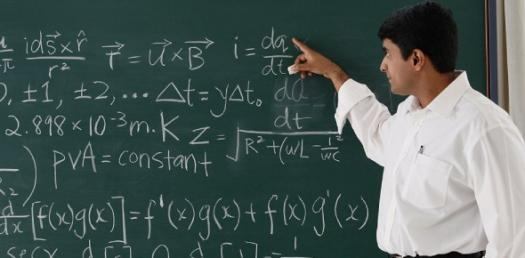# Math Test-least Common Multiple

Approved & Edited by ProProfs Editorial Team
At ProProfs Quizzes, our dedicated in-house team of experts takes pride in their work. With a sharp eye for detail, they meticulously review each quiz. This ensures that every quiz, taken by over 100 million users, meets our standards of accuracy, clarity, and engagement.
| Written by Collinjordan
C
Collinjordan
Community Contributor
Quizzes Created: 1 | Total Attempts: 3,745
Questions: 5 | Attempts: 3,758SettingsThere are two methods that can be confusing for an individual and this is finding the greatest common multiple and the least common multiple. Do you have a strong understanding on what one needs to do,so that they can get the least common multiple of some numbers? Answer the questions below. Find the least common multiple. Show your work!

• 1.

### What is 5 and 15

• A.

30

• B.

15

• C.

20

• D.

45

B. 15
Explanation
The correct answer is 15 because when you add 5 and 15 together, you get a sum of 20. The other options, 30, 20, and 45, do not result from adding 5 and 15.

Rate this question:

• 2.

• A.

30

• B.

40

• C.

90

• D.

36

D. 36
• 3.

• A.

60

• B.

35

• C.

30

• D.

65

C. 30
• 4.

### 5 and12

• A.

30

• B.

40

• C.

60

• D.

120

C. 60
Explanation
In the given sequence, the numbers are multiplied by 2 each time. Starting with 5, the next number is obtained by multiplying 5 by 2, resulting in 10. The number after 10 is obtained by multiplying 10 by 2, resulting in 20. Following the same pattern, the number after 20 is 40. Therefore, the missing number is 40.

Rate this question:

• 5.

### 8 and 9

• A.

72

• B.

40

• C.

45

• D.

70Back to top